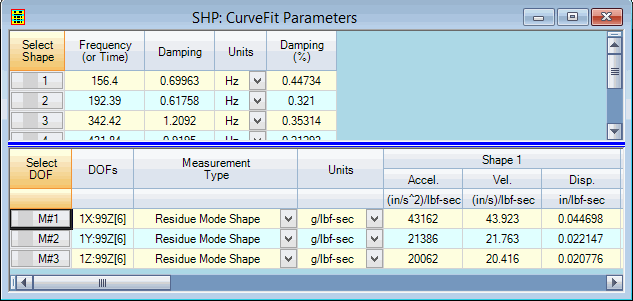# Display | Shape M#s | Accel, Vel, Disp

These commands display the shape data in the M#s spreadsheet in Acceleration, Velocity or Displacement units.These values are calculated based on the output units of the shapes. If the output units are acceleration units,

• Shapes with velocity units are calculated by dividing each shape component by the shape frequency.

• Shapes with displacement units are calculated by dividing teach shape component by the shape frequency squared.

If the output units are velocity units,

• Shapes with acceleration units are calculated by multiplying each shape component by the shape frequency.

• Shapes with displacement shapes are calculated by dividing each shape component by the shape frequency.

If the output units are displacement units,

• Shapes with velocity units are calculated by multiplying each shape component by the shape frequency.

• Shapes with acceleration units are calculated by multiplying each shape component by the shape frequency squared.